Ex 11.1

Chapter 11 Class 9 Constructions
Serial order wise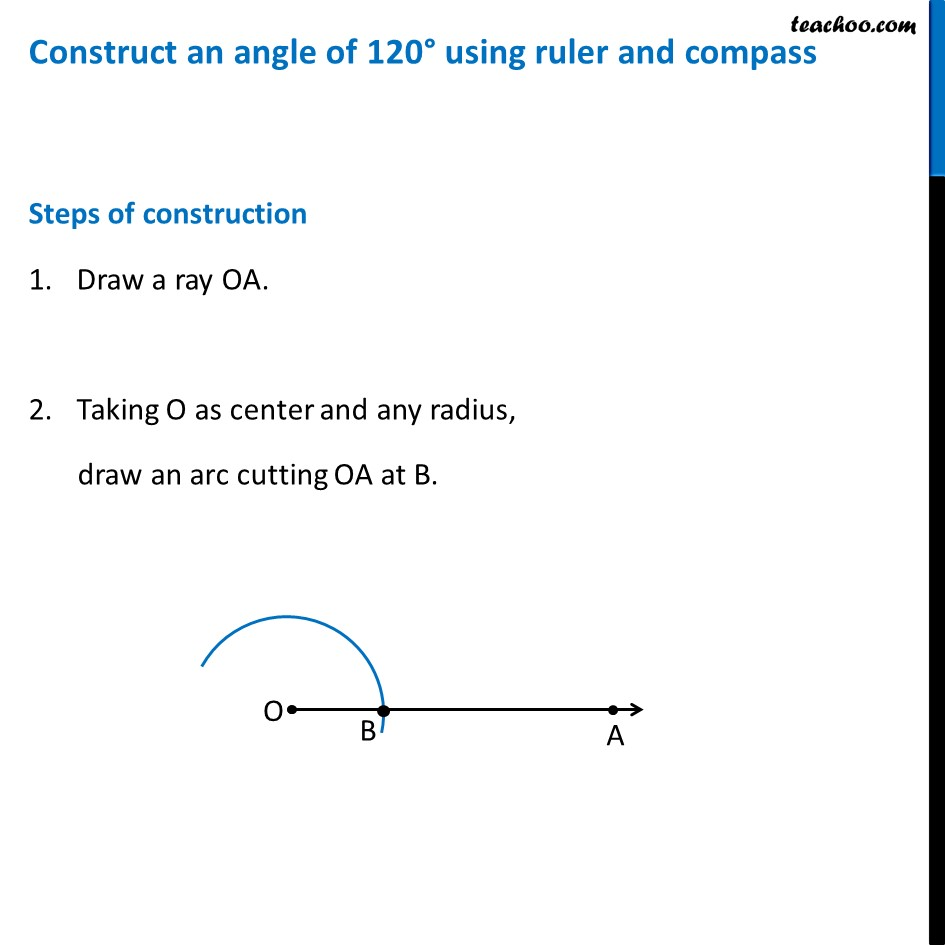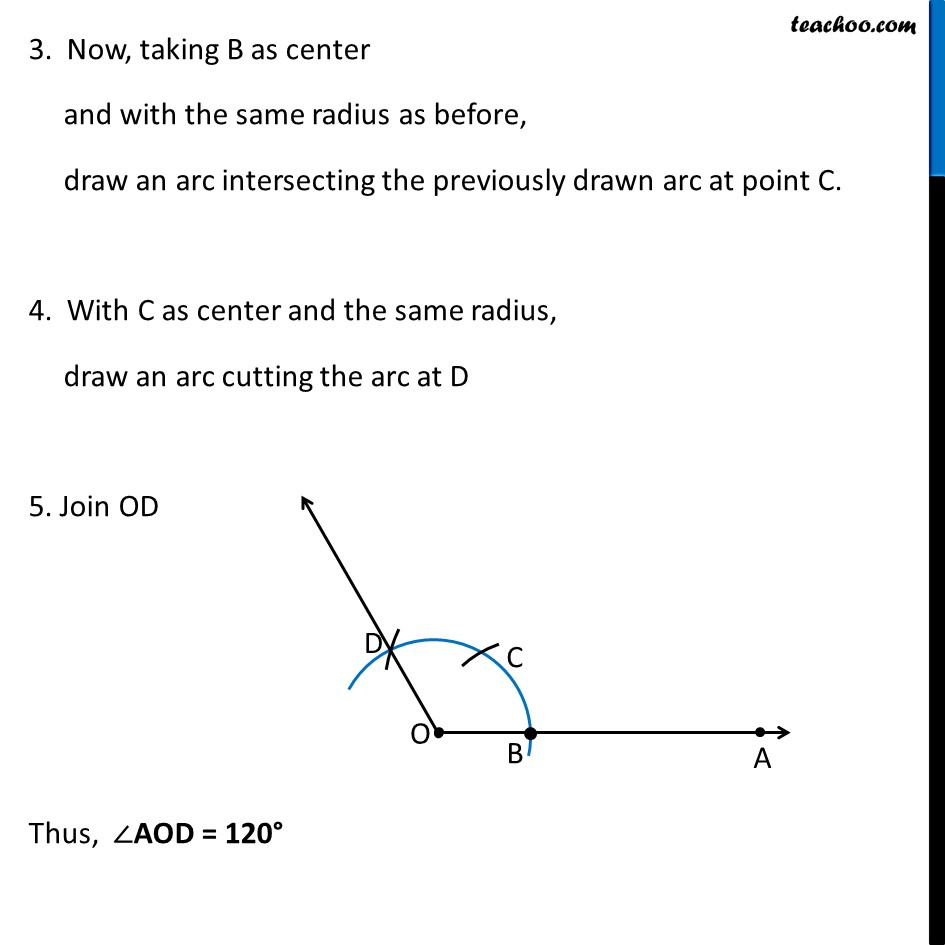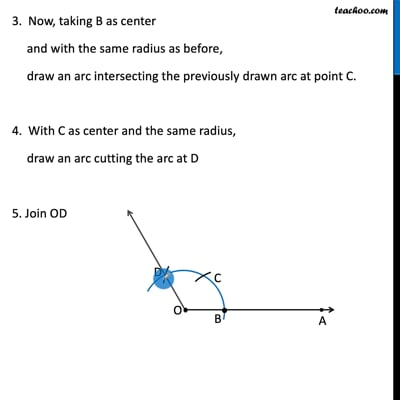This video is only available for Teachoo black users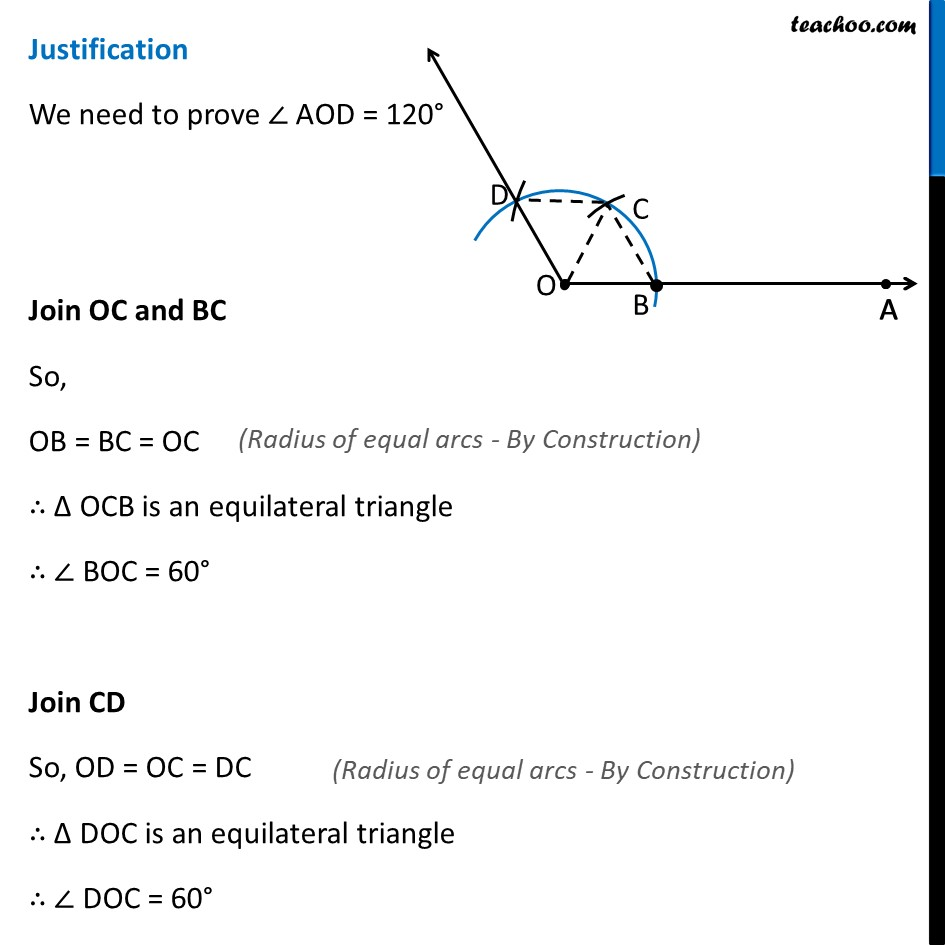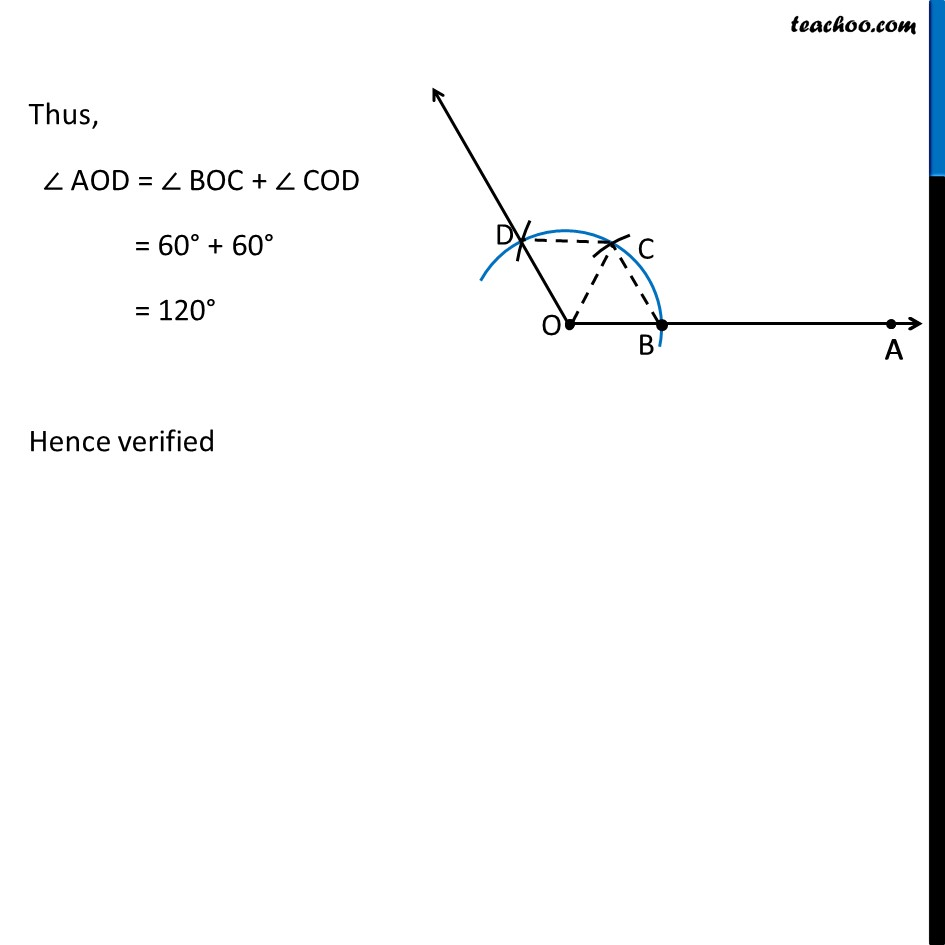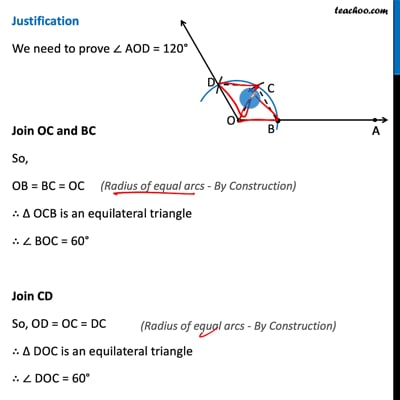This video is only available for Teachoo black users

Introducing your new favourite teacher - Teachoo Black, at only ₹83 per month

### Transcript

Construct an angle of 120° using ruler and compass Steps of construction Draw a ray OA. Taking O as center and any radius, draw an arc cutting OA at B. 3. Now, taking B as center and with the same radius as before, draw an arc intersecting the previously drawn arc at point C. 4. With C as center and the same radius, draw an arc cutting the arc at D 5. Join OD Thus, ∠AOD = 120° Justification We need to prove ∠ AOD = 120° Join OC and BC So, OB = BC = OC ∴ Δ OCB is an equilateral triangle ∴ ∠ BOC = 60° Join CD So, OD = OC = DC ∴ Δ DOC is an equilateral triangle ∴ ∠ DOC = 60° (Radius of equal arcs - By Construction) (Radius of equal arcs - By Construction) Thus, ∠ AOD = ∠ BOC + ∠ COD = 60° + 60° = 120° Hence verified